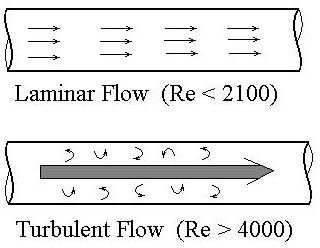# Use Reynolds Number for Pipe Flow to find Whether it is Laminar Flow or Turbulent Flow

## Introduction

For pipe flow applications, it's often important to be able to determine whether a given flow condition is laminar flow or turbulent flow. Different equations or methods of analysis often apply to laminar flow and turbulent flow conditions.

The concepts of turbulent and laminar flow are shown in the diagram in the next section. Laminar flow (also called streamline flow or viscous flow) occurs for low Reynolds number, with relatively slow flow velocity and high viscosity It is characterized by all of the fluid velocity vectors lined up in the direction of flow. Turbulent flow, on the other hand occurs at high Reynolds number, with relatively high flow velocity and low viscosity. It has point velocity vectors in all directions, although the overall flow is in one direction, along the axis of the pipe.

Practical transport of water or air in a pipe or other closed conduit is typically turbulent flow. Similarly, pipe flow of other gases or liquids with viscosity similar to water will normally be transported in conduits under turbulent flow conditions. Laminar flow will often be present with liquids of high viscosity, such as lubricating oils.

## The Reynolds NumberThe typical criterion for whether pipe flow is laminar or turbulent is the value of the Reynolds Number. The Reynolds number for pipe flow is defined as Re = DVρ/μ, where D is the pipe diameter, V is the average flow velocity in the pipe, ρ is the density of the flowing fluid and μ is the dynamic viscosity of the flowing fluid. Re is a dimensionless number. Any consistent set of units can be used for D, V, ρ and μ, and will result in Reynolds number being dimensionless. The generally accepted criteria for laminar flow and turbulent flow in terms of Re are as follows:

For Re < 2100, it is laminar flow.

For Re > 4000, it is turbulent flow.

For 2100 < Re < 4000, the flow is in the transition region.

For flow in the transition region, the flow may be either laminar or turbulent, depending upon the nature of the entrance to the pipe and the pipe wall roughness.

## Non-circular Conduits – Hydraulic Radius

Fluids also flow through non-circular, closed conduits, such as HVAC ducts or heat exchangers. In that case the definition of the Reynolds Number becomes: Re = 4RHVρ/μ. Note that pipe diameter, D, has been replaced with 4RH, where RH is the hydralic radius, defined as: RH = A/P = cross-sectional area normal to flow/wetted perimeter. For example, the hydraulic radius for a 6" by 12" rectangular furnace duct would be: RH = (0.5)(1)/[(2)(0.5) + (2)(1)] = 0.5/3 = 0.167 ft

## Example Calculations

Consider pipe flow of 1.2 cfs of water at 50oF through a 4" diameter pipe. What is the Reynolds Number for this flow? Is it laminar flow or turbulent flow?

Solution: Values for the density and viscosity of water at 50oF are needed. Such values are available in fluid mechanics or thermodynamics textbooks, handbooks and on the internet.

The values needed for this problem are: ρ = 1.94 slugs/ft3 and μ = 2.73 x 10-5 lb-sec/ft2.

The flow velocity, V, can be calculated from V = Q/A = Q/(πD2/4) = 1.2/[π(4/12)2/4] = 1.34 ft/sec.

Substituting values into Re = DVρ/μ gives Re = (1/3)(1.34)(1.94)/2.73 x 10-5, or Re = 3.17 x 104.

This value of Reynolds number is greater than 4000, so this is turbulent flow.

## This post is part of the series: Pipe Flow Calculations

Pipe flow calculations include using Reynolds number to find if the flow is laminar flow or turbulent flow. Frictional head loss can be found using the Darcy Weisbach equation and the friction factor. The entrance length for fully developed flow can be found for turbulent flow and for laminar flow.